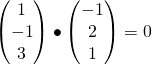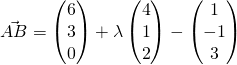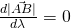# 2014 A-level H2 Mathematics (9740) Paper 1 Question 9 Suggested SolutionsAll solutions here are SUGGESTED. Mr. Teng will hold no liability for any errors. Comments are entirely personal opinions.

(i)
direction of lSince planes p and q are perpendicular, the normal of plane p is parallel to plane q.

normal of plane q, nThus, the cartesian equation of q is.

(ii)
From GC, equation of m is.

(iii)
Letfor someTo find the minimum distance, differentiatewith respect to,At minimum distance,,Thus, required coordinates is.

The first and second part are standard tutorial questions that students should be able to handle. Students need to realise the use of GC in finding line of intersection is most effective. For (iii), there are several methods, students can complete the square to find the turning point. Alternatively, students can graph the expression found on GC and find the minimum. They find the same answers too, but it will not be exact. As for students that are keen on following the “otherwise” approach, they can use the foot of perpendicular from A to B on m. Lastly, since the question asked for coordinates, students are expected to give in coordinate form.

•Jq

For 9i shouldn’t the vector be (-5 10 -10)

•Jq

Sorry I’m careless

Not readable? Change text.